78 school students graduate from mathematics or physics. There are three times more students who graduate from mathematics and do not graduate from physics than those who graduate from physics and do not graduate from mathematics. 69 students graduate from mathematics. How many students graduate from mathematics and physics?

p =  42

### Step-by-step explanation:

m+f+p=78
m=3f
m+p=69

m+f+p=78
m=3•f
m+p=69

f+m+p = 78
3f-m = 0
m+p = 69

f = 9
m = 27
p = 42

Our linear equations calculator calculates it.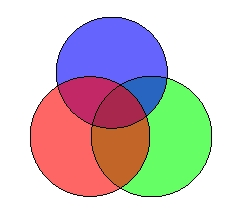Did you find an error or inaccuracy? Feel free to write us. Thank you!Tips to related online calculators
Do you have a system of equations and looking for calculator system of linear equations?

## Related math problems and questions:

• Christmas or EasterPlease calculate this example by the Venn equation. They asked 73 students whether they like Christmas or Easter. 34 of them like one of the holidays. 39 loves Easter. There are twice as many students who wish both holidays as those who only love Easter.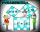Two fifth-graders teams competing in math competitions - in Mathematical Olympiad and Pytagoriade. Of the 33 students competed in at least one of the contest 22 students. Students who competed only in Pytagoriade were twice more than those who just compet
• Alcohol 2Two types of alcohol one 63% and second 75% give 20 liters of 69% alcohol. How many liters of each type are in the mixture?
• Glasses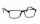There are 36 pupils in the class. Nine girls wear glasses. Boys with glasses are five less than girls without glasses. Boys without glasses are two times more than girls without glasses. How many boys and how many girls?
• Operations with sets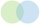The set B - A has twice as fewer elements than the set A - B and four times fewer elements than the set A ∩ B. How many times more elements does the set A have than the set B?
• Columns of two and threeWhen students in one class stand in columns of two, there is none left. When he stands in columns of three, there is one student left. There are 5 more double columns than three columns. How many students are in the class?
• SchoolsThree schools are attended by 678 pupils. To the first attend 21 students more and to the third 108 fewer students than to second school. How many students attend the schools?
• Percentages and numbers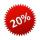How many percent is number 69 smaller than number 276?
• At theAt the presentation of the travelers came three times as many men than women. When eight men left with their partners, there were five times more men than women at the presentation. How many were men and women originally?
• Employees1116 people are working in three factory halls. In the first one, there are 18% more than the third, and 60 persons more than the second. How many employees work in individual halls?
• Two sets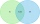Suppose Set B contains 69 elements and the total number elements in either Set A or Set B is 124. If the Sets A and B have 29 elements in common, how many elements are contained in set A?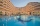Up to 48 rooms, some of which are triple and some quadruple, accommodated 173 people so that all beds are occupied. How many triple and how many quadruple rooms were there?On the meadow grazing horses, cows, and sheep, together with less than 200. If cows were 45 times more, horses 60 times more, and sheep 35 times more than there are now, their numbers would equally. How many horses, cows, and sheep are on the meadow toget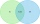University students chose a foreign language for the 1st year. Of the 120 enrolled students, 75 chose English, 65 German, and 40 both English and German. Using the Venn diagram, determine: - how many of the enrolled students chose English only - how manyIf the fifth-grade class left 20% of students and in the seventh grade were added two pupils, in the eighth one pupil, the number has not changed in the ninth grade, but it is now tenth students less than it was in the fifth grade. How many pupils are in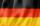Of the 35 students of the class were 7 on holiday in Germany and just as much in Italy. 5 students visited Austria. In none of these countries was 21 students, all three visited by one student. In Italy and Austria were 2 students and in Austria and Germa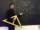There is 31 students in a class. From mathematics was'nt worse mark than 2. Average mark in mathematics was 2. How many students have mark 1 and how many mark 2?